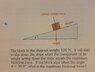# What is the maximum frictional force?

• Shaye

#### Shaye

Homework Statement
The block in the diagram weighs 120N. It will start to slip down the slope when the component of it's weight acting down the slop equals the maximum frictional force. If the block slips when the angle Θ = 35 degrees what is the maximum frictional force?
Relevant Equations
Sin(Θ)*Opposite = Hypotenuse and F=Ma
In the attachment I am supposed to calculate the maximum frictional force of a block on a 35 degree angle incline (that is the point at which the force acting opposite frictional force is highest)

I make it out to be sin(35)*120N = 69N but the book says 675N and it gives it as cost(55)*120N*9.81. But given F=Ma how so?

I don't understand this or something has slipped my mind. The diagonal force component (hypotenuse) should be 69N because the veridical component is 120N.

Thanks

#### Attachments

•20201107_163943.jpg
58.3 KB · Views: 67

•